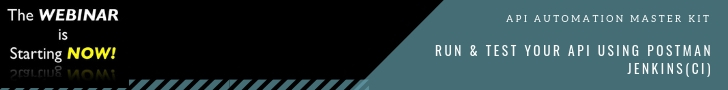# Reducing Risks in Machine Learning: Top Tests You Can Conduct20Sep, 2018

Machine learning has been a subject of interest because of its ability to use the information to solve complex problems, including facial recognition, handwriting detection etc. At times, this is done by having varied tests fitted into the machine learning algorithms, such as tests establishing thresholds, formulating a statistical hypothesis, minimizing mean squared errors and so on. Machine learning algorithms have an ability to minimize errors over the course of time and learn from past mistakes.Given that we have problems including unstable data, overfitted models, under fitted models, and undetermined future buoyancy, what can be done? There are certain general techniques and guidelines, called heuristics, which we can write into the tests for mitigating the vulnerabilities of these problems arising. These are given in detail below.## Check Fit by Cross-Validating

In penetration testing terminology, cross-validation is defined as a method to split all your data into two categories- training and validation.

• Training data is utilized to build the learning model of the machine
• Validation data is required to validate that the model does what is expected of it to be done. It also increases our ability to determine underlying errors and detect them as prevailing in the model.

Training has a special meaning in the world of machine learning. Since the algorithms of machine learning focus on mapping the previous observations with the results. The algorithms usually learn from the data collected and hence, without the initial set of data, the algorithms are of no use. In certain cases, swapping training and validation interchangeably helps in increasing the total tests number. This can be achieved by splitting up the entire data into 2; at the initial step the first set would be used for training and second set for validating, and then both would be swapped for the next test. Based upon the quantity of data available, it can be split into considerably smaller data sets and cross-validation can be performed that way. If sufficient data is available, cross-validation can be split into an undefined bulk of sets.

## Seam Testing to Lessen Unstable Data

Seams can be defined simply as the integration points between parts of the code base. Under legacy coding, at times, a tester is given a string of code where a tester can sufficiently predict what will happen when that code is fed with a set of data points, although not knowing what it does internally to arrive at the result. Machine learning is somewhat akin to legacy coding, though the algorithms are not exactly legacy code. Hence, much like legacy code, machine learning algorithms must be treated like a black box where data flows in and flows out of the algorithm. These two seams can, therefore, be tested by unit testing data inputs and outputs in order to ensure they are valid within the specified set of preferences.

A good example for above would be testing a neural network. In cases where data that is yielded to the neural network stands between 0 and 1 and the outcome is required to be 1, when the data sums to 1, it would mean it is modelling a percentage. For example, if you possess 3 spinners and 2 widgets, the array of data, in this case, would be 3/5 spinners and 2/5 widgets. In this way, seam testing defines interfaces between pieces of code. It is crucial to note that the more complex the data gets, the more important these seam tests are.## Use Precision and Recall to Monitor for Future Shifts

Precision and recall basically are two ways to monitor the potential of the implementation of machine learning. Precision is actually a metric which monitors the rate of the true positives. For instance, a 4/7 precision would imply that 4 out of the total seven submitted to a user were correct.  The recall is generally defined as a ratio of true positives to the summation of true positives and false negatives.

User input is required for calculating precision and recalling. This encloses the learning curve and enhances data over a period of time because of information getting fed back after getting misclassified. Netflix, for example, demonstrates this by showing star rating, which it predicts you, would give to a particular movie depending upon your movie-watching history. If you do not agree with this and rank it adversely or show that you are not actually interested, Netflix will feed it back into the model for later predictions.

##Testing the Speed of Training to Reduce Overfitting Risk

Simplicity is a great virtue while modelling data and the simpler solution is usually the better one.

In testing terms, this means that one should not overfit his data since overfitted models generally just memorize the amount of data given to them. In cases where a simpler solution can be identified, you will notice the patterns versus parsing out the erstwhile data.

One of the better proxies for complexity in a traditional machine learning model is the ability to quickly train the model. For instance, when there are two varying approaches to train a machine learning model, one taking three hours and another taking mere 30 minutes, the one taking less time is usually the better, irrespective of other things being unequal. A workable approach, therefore, is to wrap a benchmark across the code to identify if the model is getting slower over the period of time.

Give us 30 minutes and we will show you how many millions you can save by outsourcing software testing. Make Your product quality top notch. Talk to us to see how